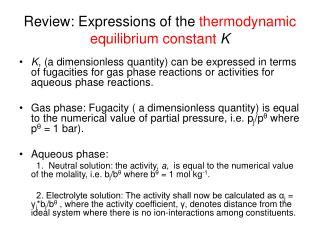DownloadDownload PresentationReview: Expressions of the thermodynamic equilibrium constant K

# Review: Expressions of the thermodynamic equilibrium constant K

Download Presentation## Review: Expressions of the thermodynamic equilibrium constant K

- - - - - - - - - - - - - - - - - - - - - - - - - - - E N D - - - - - - - - - - - - - - - - - - - - - - - - - - -
##### Presentation Transcript

1. Review: Expressions of the thermodynamic equilibrium constantK • K, (a dimensionless quantity) can be expressed in terms of fugacities for gas phase reactions or activities for aqueous phase reactions. • Gas phase: Fugacity ( a dimensionless quantity) is equal to the numerical value of partial pressure, i.e. pj/pθ where pθ = 1 bar). • Aqueous phase: 1. Neutral solution: the activity, a, is equal to the numerical value of the molality, i.e. bj/bθ where bθ = 1 mol kg-1. 2. Electrolyte solution: The activity shall now be calculated as αj = γj*bj/bθ , where the activity coefficient, γ, denotes distance from the ideal system where there is no ion-interactions among constituents.

2. The activities of solids and pure liquid are equal to 1 • α(solid) = 1 and α(pure liquid) = 1 (!!!) • Illustration: Express the equilibrium constant for the heterogeneous reaction NH4Cl(s) ↔ NH3(g) + HCl(g) • Solution: In term of fugacity (i.e. thermodynamic equilibrium constant): Kp = In term of molar fraction: Kx =

3. Estimate reaction compositions at equilibrium • Example 1: Given the standard Gibbs energy of reaction H2O(g) → H2(g) + 1/2O2(g) at 2000K is + 135.2 kJ mol-1, suppose that steam at 200k pa is passed through a furnace tube at that temperature. Calculate the mole fraction of O2 present in the output gas stream. • Solution: (details will be discussed in class) lnK = - (135.2 x 103 J mol-1)/(8.3145 JK-1mol-1 x 2000K) = - 8.13037 K = 2.9446x10-4 K = Ptotal = 200Kpa assuming the mole fraction of O2 equals x PO2 = x* Ptotal, PH2 = 2(x*Ptotal) PH2O = Ptotal – PO2 – PH2 = (1-3x)Ptotal

4. Equilibria in biological systems:Standard reaction Gibbs energy for biochemical systems • Biological standard state: pH = 7. • For a reaction: A + vH+(aq)↔ P ΔrG = ΔrGθ + RT = ΔrGθ + RT the first two terms of the above eq. form ΔrG‡ ΔrG‡ = ΔrGθ + 7vRTln10, A better practice is to transfer the above eq into ΔrG‡ = ΔrGθ - 7vRTln10, and then recognizes v is the stoichiometric number of H+.

5. Example: For a particular reaction of the form A → B + 2H+ in aqueous solution, it was found that ΔrGθ = 20kJ mol-1 at 28oC. Estimate the value of ΔrG‡. • Solution: ΔrG‡ = ΔrGθ - 7vRTln10 here the stoichiometric number of H+ is 2, i.e. v = 2 ΔrG‡ = 20 kJ mol-1 - 7(2)(8.3145x10-3 kJ K-1mol-1) x(273+ 28K)ln10 = 20 kJ mol-1 – 80.676 kJ mol-1 = -61 kJ mol-1 (Notably, when measured with the biological standard, the standard Gibbs energy of reaction becomes negative! A transition from endergonic to exergonic process )

6. Molecular Interpretation of equilibrium Two factors affect the thermodynamic equilibrium constant: (1) Enthalpy, and (2) Entropy. Boltzmann distribution is independent of the nature of the particle.

7. The response of equilibria to reaction conditions • Equilibria may respond to changes in pressure, temperature, and concentrations of reactants and products. • The equilibrium constant is not affected by the presence of a catalyst.

8. How equilibria respond to pressure • The thermodynamic equilibrium constant K is a function of the standard reaction Gibbs energy, ΔrGθ . • Standard reaction Gibbs energy ΔrGθ is defined at a single standard pressure and thus is independent of the pressure used in a specific reaction. • The equilibrium constant is therefore independent of reaction pressure. Such a relationship can be expressed as:

9. Although the thermodynamics equilibrium constant K is independent of pressure, it does not mean that the equilibrium composition is independent of the pressure!!! • Example: Consider a gas reaction 2A(g) ↔ B(g) assuming that the mole fraction of A equals xA at quilibrium, then xB = 1.0 – xA, because K does not change, xA must change in response to any variation in Ptotal!!!

10. Le Chatelier’s Principle • A system at equilibrium, when subject to a disturbance, responds in a way that tends to minimize the effect of the disturbance. • The above statement suggests that if the total pressure of a system is increased, the system will shift to the direction that will have smaller number of molecules, i.e. smaller pressure. • 3H2(g) + N2(g) ↔ 2NH3(g).

11. Example: Predict the effect of an increase in pressure on the Haber reaction, 3H2(g) + N2(g) ↔ 2NH3(g). • Solution: According to Le Chatelier’s Principle, an increase in pressure will favor the product. prove: Therefore, to keep the thermodynamic equilibrium constant K unchanged, the equilibrium mole fractions Kx must change by a factor of 4 if the pressure ptotal is doubled.

12. The response of equilibria to temperature • According to Le Chatelier’s Principle: Exothermic reactions: increased temperature favors the reactants. Endothermic reactions: increased temperature favors the products. • The van’t Hoff equation: (a) (7.23a) (b) (7.23b)

13. Differentiate lnK with respect to temperature Using Gibbs-Helmholtz equation (eqn 3.53 8th edition) thus Because d(1/T)/dT = -1/T2: Derivation of the van’t Hoff equation:

14. For an exothermic reaction, ΔrHθ < 0, thus , suggesting that increasing the reaction temperature will reduce the equilibrium constant.